# INTERVAL VELOCITY AND NULL SPACE

A central problem in reflection seismology is building the velocity as a function of depth (or vertical travel time) starting from certain measurements. The measurements are described elsewhere (BEI for example). They amount to measuring the integral of the velocity squared from the surface down to the reflector. The integral gives the RMS (root-mean-square) velocity. Although good quality echos may arrive often, they rarely arrive continuously for all depths. Good information is interspersed unpredictably with poor information. Luckily we can also estimate the data quality by the coherency'' or the stack energy''. In summary, what we get from observations and preprocessing are two functions of travel-time depth: (1) the velocity (squared) and integrated from the surface, and (2) a measure of the quality of the integrated velocity estimate. Some definitions:is the data vector given to us by the preprocessing. Its components range over the vertical traveltime depth,and its component values contain the scaled RMS velocity squaredwhere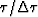is the index on the time axis.is a diagonal matrix along which we lay the given measure of data quality. We will use it as a weighting function.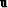is the vector we seek. Its components range over the vertical traveltime depth,and its component values contain the local velocity (called the interval velocity) squared.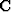is the matrix of causal integration, a lower triangular matrix of ones.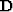is the matrix of causal differentiation, namely,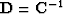.

From these definitions, under the assumption of a stratified earth with horizontal reflectors (and no multiple reflections) the theoretical interval velocity enables us to define the theoretical RMS velocity by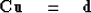(1)
Our data-fitting goal is to minimize the residual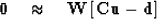(2)

To find the interval velocity where there is no data (where the stack power vanishes) we have the model damping'' goal to minimize the wiggliness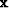of the (squared) interval velocity.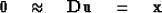(3)

We precondition'' these two goals by changing the optimization variable from interval velocity squaredto its wiggliness.Substitutinggives the two goals expressed as a function of wiggliness.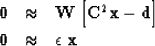(4) (5)